# CHAPTER3 PROBLEM SOLVING AND SEARCH CONT 2 Searching

• Slides: 27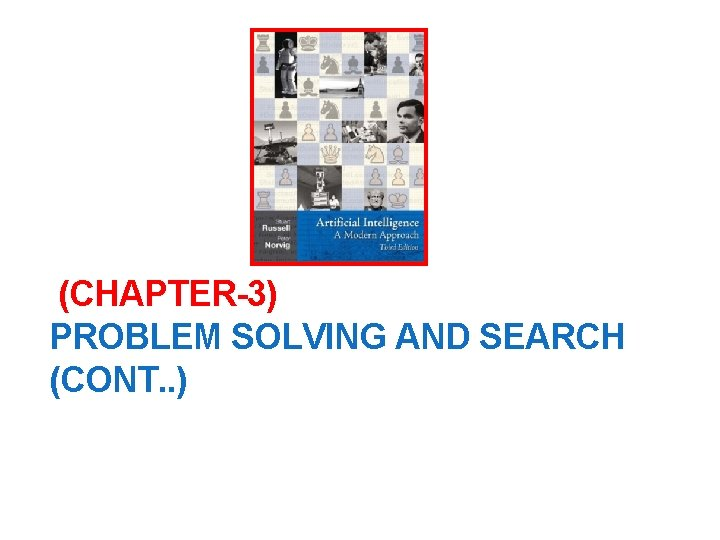(CHAPTER-3) PROBLEM SOLVING AND SEARCH (CONT. . )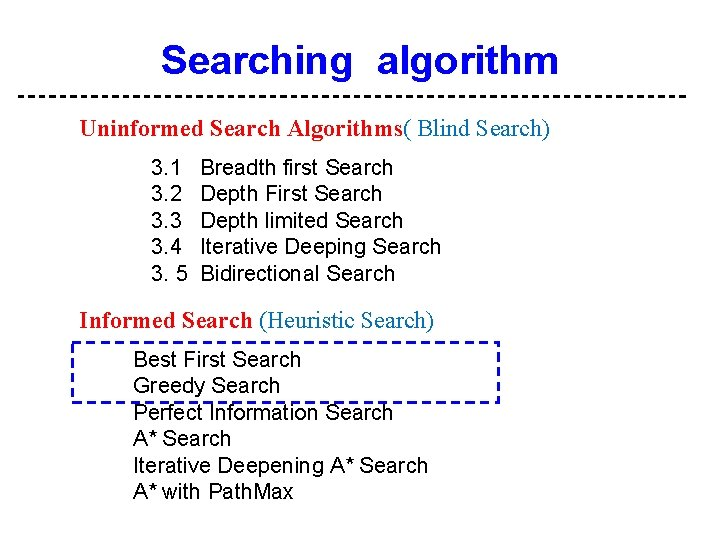2 Searching algorithm Uninformed Search Algorithms( Blind Search) 3. 1 3. 2 3. 3 3. 4 3. 5 Breadth first Search Depth First Search Depth limited Search Iterative Deeping Search Bidirectional Search Informed Search (Heuristic Search) Best First Search Greedy Search Perfect Information Search A* Search Iterative Deepening A* Search A* with Path. Max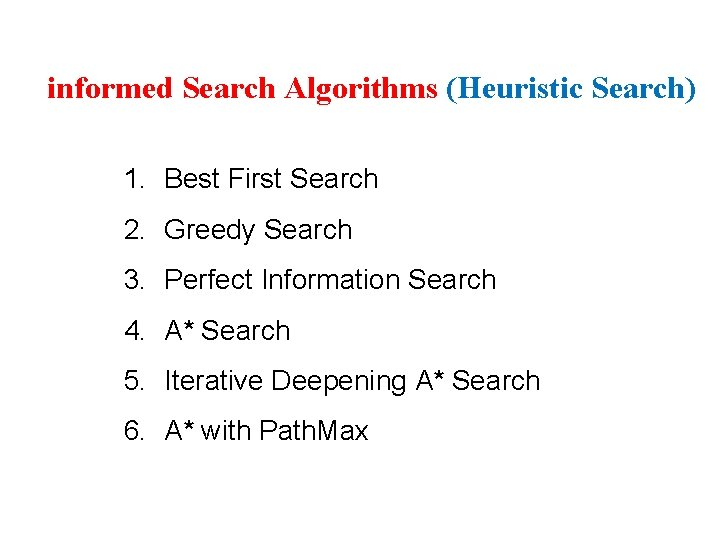3 informed Search Algorithms (Heuristic Search) 1. Best First Search 2. Greedy Search 3. Perfect Information Search 4. A* Search 5. Iterative Deepening A* Search 6. A* with Path. Max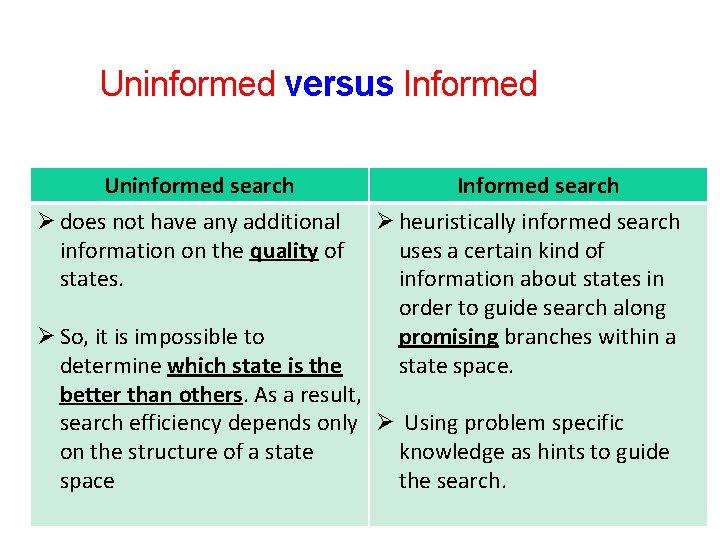4 Uninformed versus Informed Uninformed search Ø does not have any additional information on the quality of states. Informed search Ø heuristically informed search uses a certain kind of information about states in order to guide search along promising branches within a state space. Ø So, it is impossible to determine which state is the better than others. As a result, search efficiency depends only Ø Using problem specific on the structure of a state knowledge as hints to guide space the search.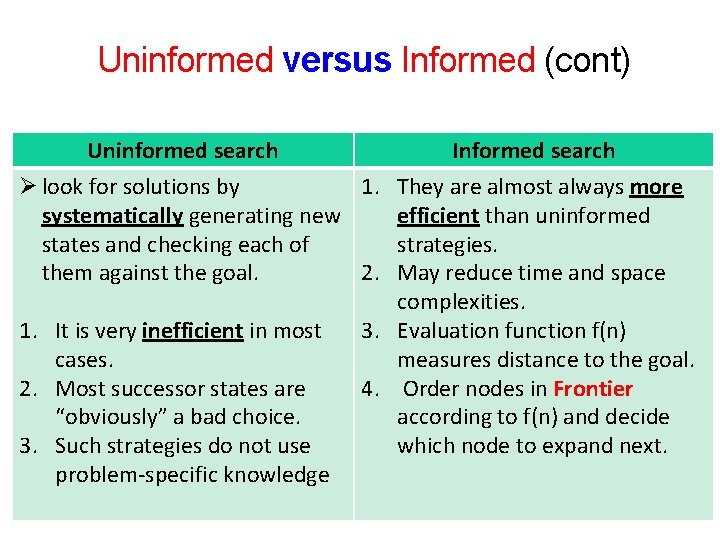5 Uninformed versus Informed (cont) Uninformed search Informed search Ø look for solutions by 1. They are almost always more systematically generating new efficient than uninformed states and checking each of strategies. them against the goal. 2. May reduce time and space complexities. 1. It is very inefficient in most 3. Evaluation function f(n) cases. measures distance to the goal. 2. Most successor states are 4. Order nodes in Frontier “obviously” a bad choice. according to f(n) and decide 3. Such strategies do not use which node to expand next. problem-specific knowledge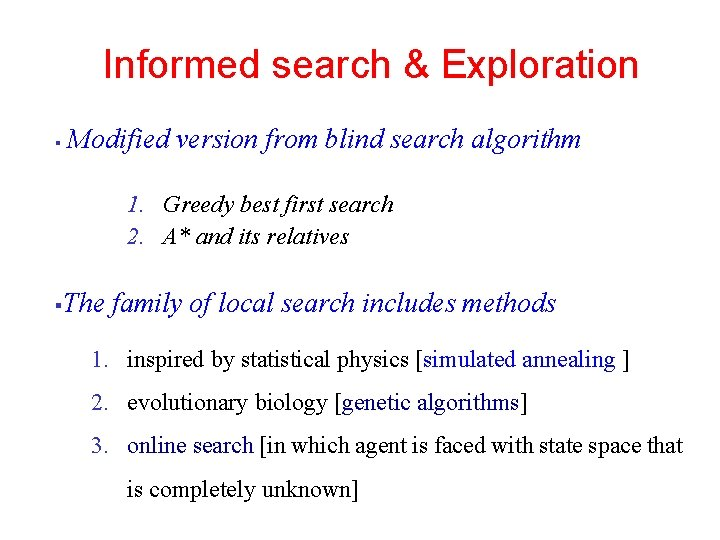6 Informed search & Exploration § Modified version from blind search algorithm 1. Greedy best first search 2. A* and its relatives § The family of local search includes methods 1. inspired by statistical physics [simulated annealing ] 2. evolutionary biology [genetic algorithms] 3. online search [in which agent is faced with state space that is completely unknown]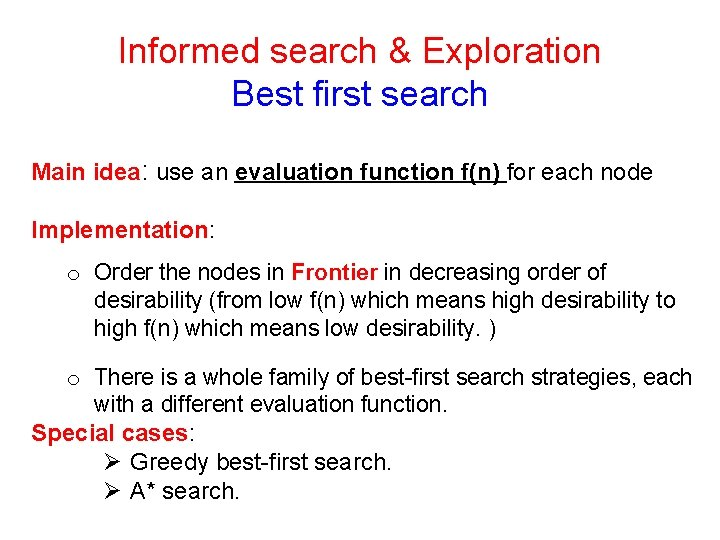7 Informed search & Exploration Best first search Main idea: use an evaluation function f(n) for each node Implementation: o Order the nodes in Frontier in decreasing order of desirability (from low f(n) which means high desirability to high f(n) which means low desirability. ) o There is a whole family of best-first search strategies, each with a different evaluation function. Special cases: Ø Greedy best-first search. Ø A* search.8 Best-first search Algorithm 1 -greedy best first search Ø Tries to expand the node that is closest to the goal o Use straight line distance ex : h. SLD(IN(Arad))=366 [note that the values of fn h. SLD cannot be computed from the problem description itself]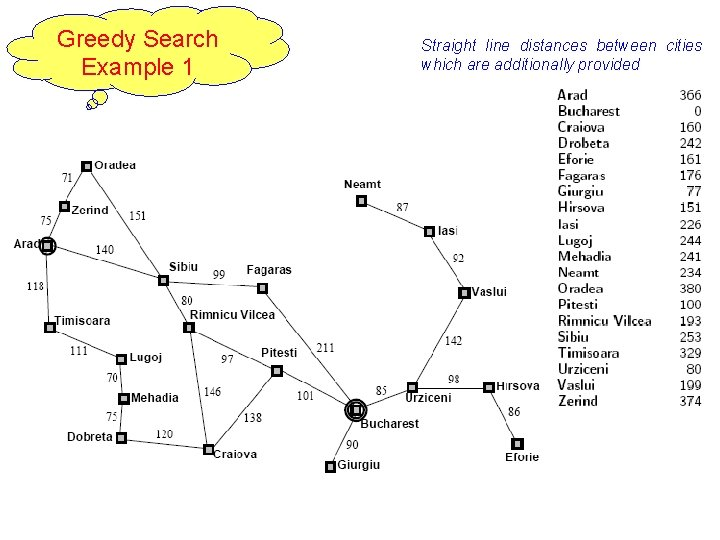Greedy Search Example 1 9 Straight line distances between cities which are additionally provided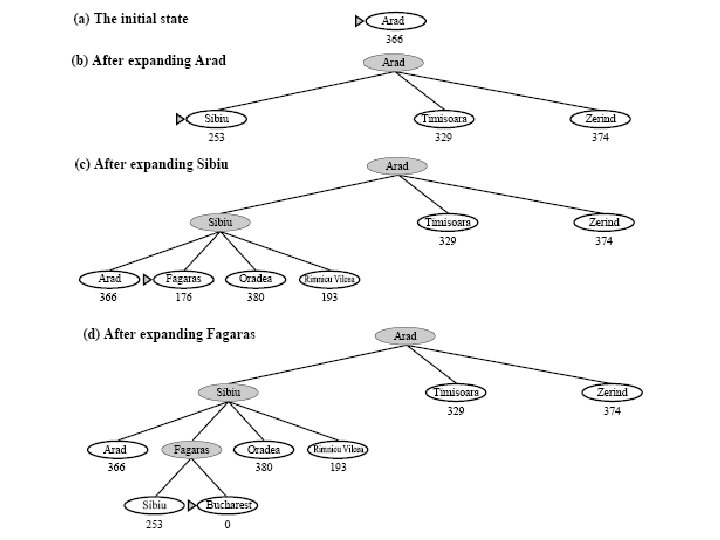11 The greedy best first search using h. SLD finds a solution without ever expanding a node that is not on solution path, hence its cost is minimal This show why the algorithm is called greedy [at each step it tries to get as close to goal as it can]Greedy Search Example 2 12 Straight line distances between cities which are additionally provided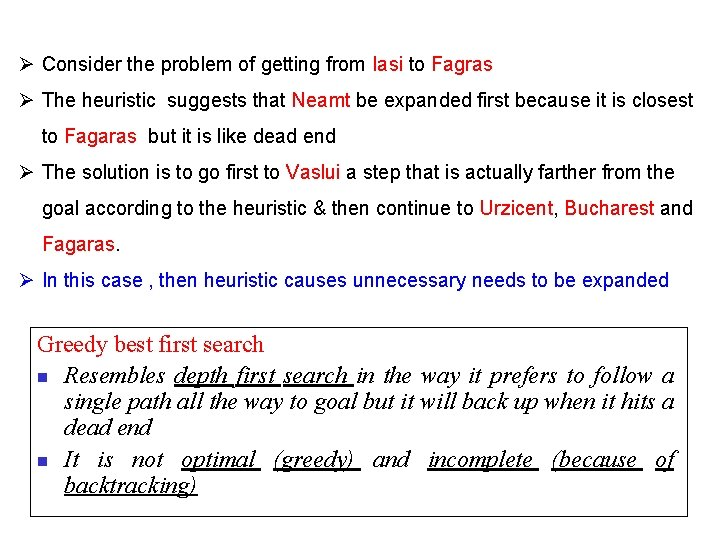13 Ø Consider the problem of getting from Iasi to Fagras Ø The heuristic suggests that Neamt be expanded first because it is closest to Fagaras but it is like dead end Ø The solution is to go first to Vaslui a step that is actually farther from the goal according to the heuristic & then continue to Urzicent, Bucharest and Fagaras. Ø In this case , then heuristic causes unnecessary needs to be expanded Greedy best first search n Resembles depth first search in the way it prefers to follow a single path all the way to goal but it will back up when it hits a dead end n It is not optimal (greedy) and incomplete (because of backtracking)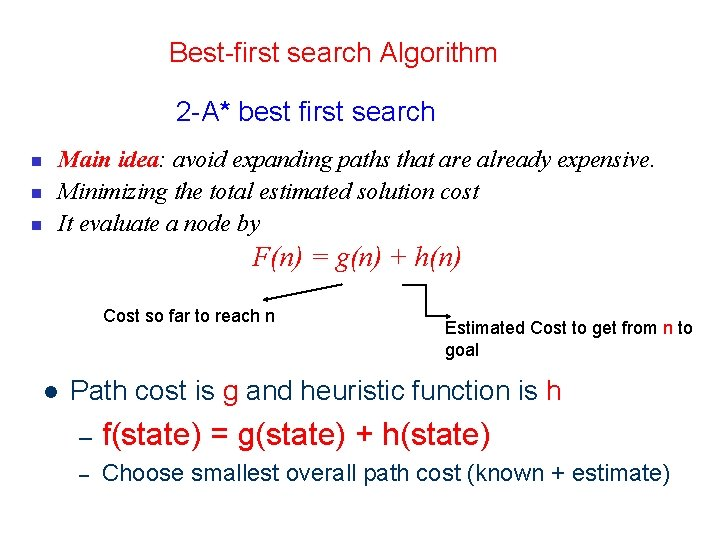14 Best-first search Algorithm 2 -A* best first search n n n Main idea: avoid expanding paths that are already expensive. Minimizing the total estimated solution cost It evaluate a node by F(n) = g(n) + h(n) Cost so far to reach n l Estimated Cost to get from n to goal Path cost is g and heuristic function is h – f(state) = g(state) + h(state) – Choose smallest overall path cost (known + estimate)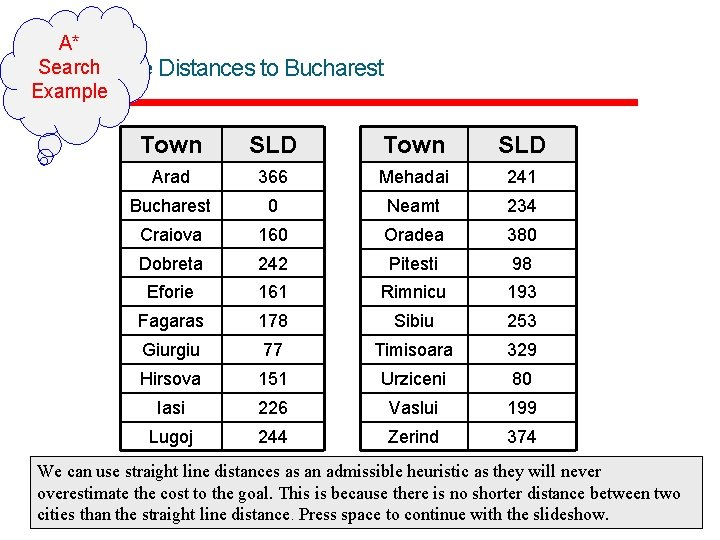15 A* Search Line Distances to Bucharest Straight Example Town SLD Arad 366 Mehadai 241 Bucharest 0 Neamt 234 Craiova 160 Oradea 380 Dobreta 242 Pitesti 98 Eforie 161 Rimnicu 193 Fagaras 178 Sibiu 253 Giurgiu 77 Timisoara 329 Hirsova 151 Urziceni 80 Iasi 226 Vaslui 199 Lugoj 244 Zerind 374 We can use straight line distances as an admissible heuristic as they will never overestimate the cost to the goal. This is because there is no shorter distance between two cities than the straight line distance. Press space to continue with the slideshow.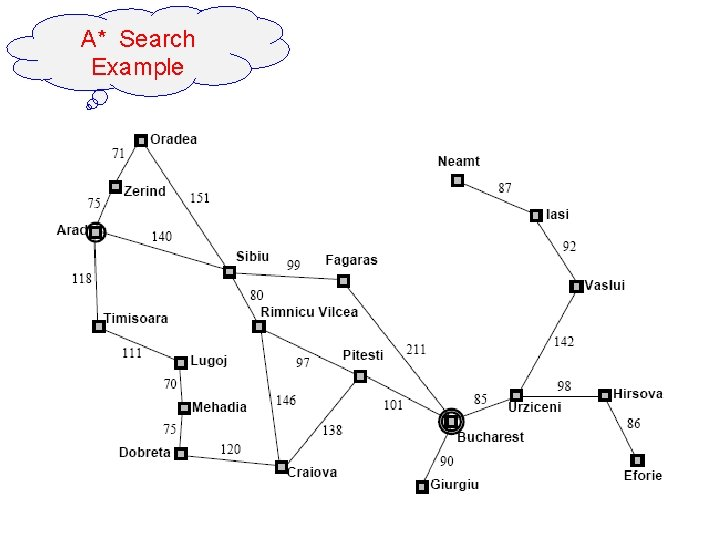A* Search Example 16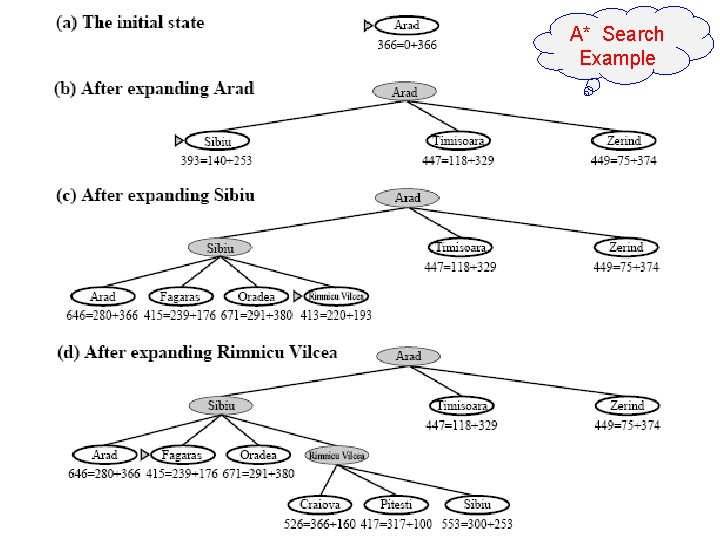A* Search Example 17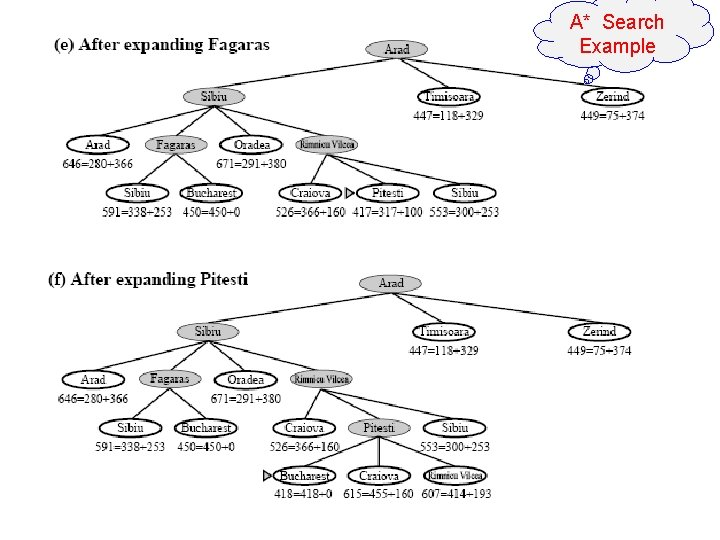A* Search Example 18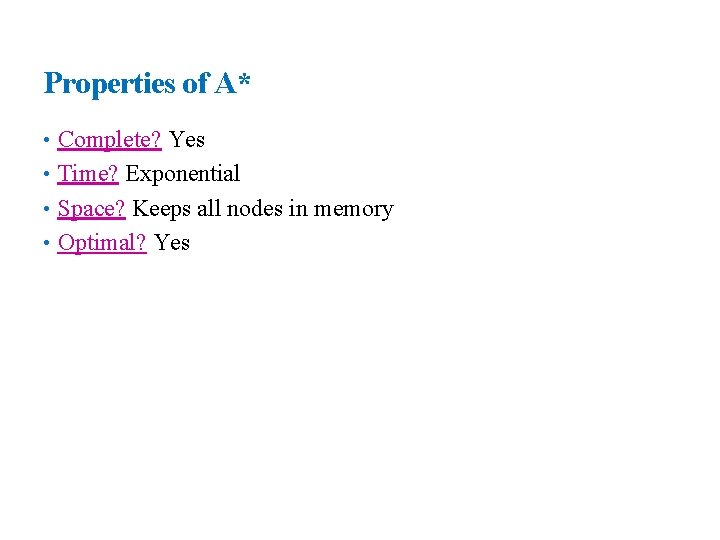19 Properties of A* • Complete? Yes • Time? Exponential • Space? Keeps all nodes in memory • Optimal? Yes20 Examples21 Shortest paths • Find a shortest path from v 0 to v 3? ? ? • Can the greedy method solve this problem? ? ? • The shortest path: 1 + 2 + 4 = 7.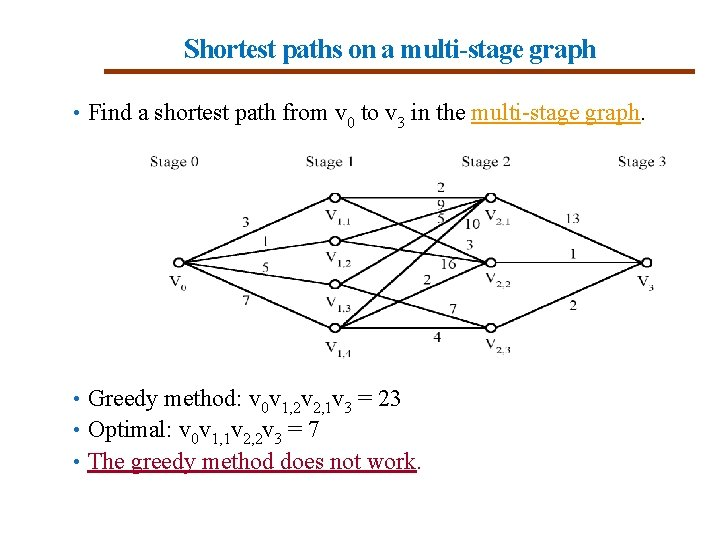22 Shortest paths on a multi-stage graph • Find a shortest path from v 0 to v 3 in the multi-stage graph. • Greedy method: v 0 v 1, 2 v 2, 1 v 3 = 23 • Optimal: v 0 v 1, 1 v 2, 2 v 3 = 7 • The greedy method does not work.23 Admissible heuristics (accepted evaluation function) E. g. , for the 8 -puzzle: • h 1(n) = number of misplaced tiles[tiles in wrong places) • h 2(n) = total Manhattan distance [how many moves to reach right place] (i. e. , no. of squares from desired location of each tile) Start state • h 1(S) = ? • h 2(S) = ? Goal state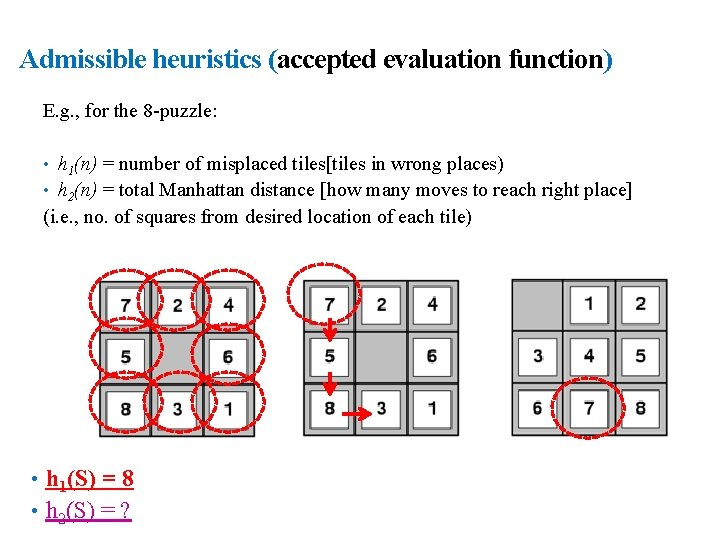24 Admissible heuristics (accepted evaluation function) E. g. , for the 8 -puzzle: • h 1(n) = number of misplaced tiles[tiles in wrong places) • h 2(n) = total Manhattan distance [how many moves to reach right place] (i. e. , no. of squares from desired location of each tile) • h 1(S) = 8 • h 2(S) = ?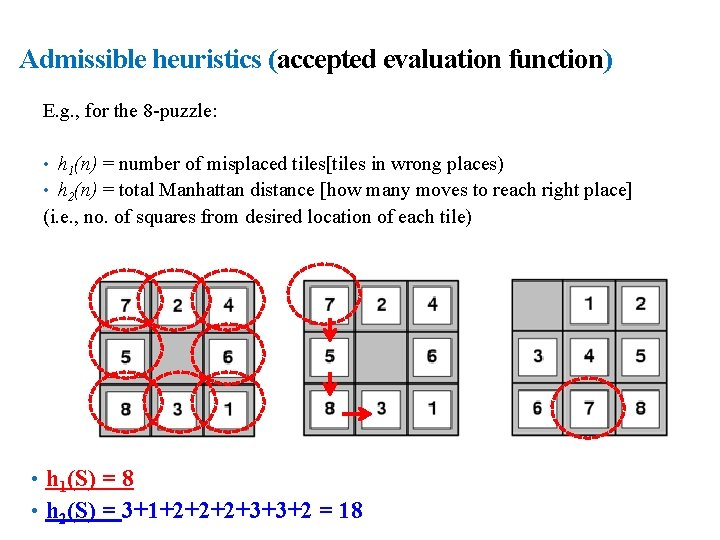25 Admissible heuristics (accepted evaluation function) E. g. , for the 8 -puzzle: • h 1(n) = number of misplaced tiles[tiles in wrong places) • h 2(n) = total Manhattan distance [how many moves to reach right place] (i. e. , no. of squares from desired location of each tile) • h 1(S) = 8 • h 2(S) = 3+1+2+2+2+3+3+2 = 1826 Dominance (which is better) • If h 2(n) ≥ h 1(n) for all n (both admissible) • then h 2 dominates h 1 • h 2 is better for search • Typical search costs (average number of nodes expanded): • d=12 IDS = 3, 644, 035 nodes A*(h 1) = 227 nodes A*(h 2) = 73 nodes • d=24 IDS = too many nodes A*(h 1) = 39, 135 nodes A*(h 2) = 1, 641 nodes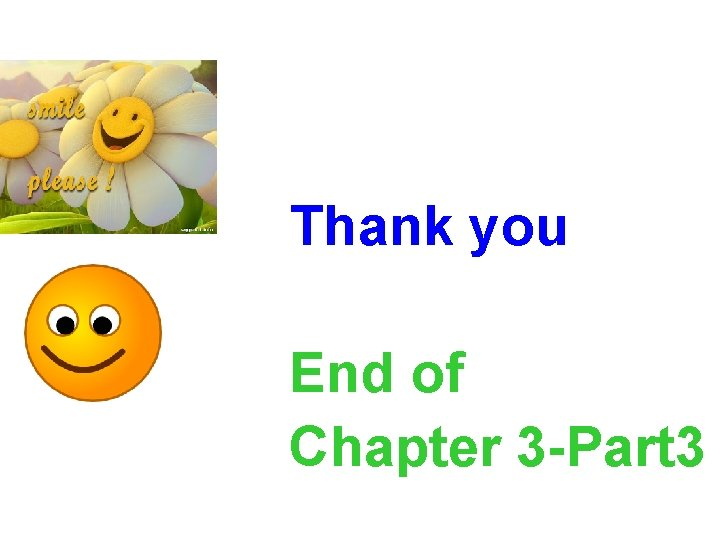27 Thank you End of Chapter 3 -Part 3